International
Tables for
Crystallography
Volume D
Physical properties of crystals
Edited by A. Authier

International Tables for Crystallography (2006). Vol. D, ch. 1.1, pp. 4-5

## Section 1.1.1.4. Symmetry of the matrix of physical properties

A. Authiera*

aInstitut de Minéralogie et de la Physique des Milieux Condensés, Bâtiment 7, 140 rue de Lourmel, 75015 Paris, France
Correspondence e-mail: aauthier@wanadoo.fr

#### 1.1.1.4. Symmetry of the matrix of physical properties

| top | pdf |

If parameter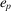varies by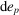, the specific energy varies by du, which is equal to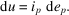We have, therefore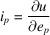and, using (1.1.1.5),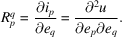Since the energy is a state variable with a perfect differential, one can interchange the order of the differentiations:Since p and q are dummy indices, they may be exchanged and the last term of this equation is equal to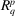. It follows that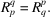Matrices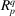and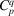are therefore symmetric. We may draw two important conclusions from this result:

 (i) The submatrices associated with the principal properties are symmetric with respect to interchange of the indices related to the causes and to the effects: these properties are represented by symmetric tensors. For instance, the dielectric constant and the elastic constants are represented by symmetric tensors of rank 2 and 4, respectively (see Section 1.1.3.4). (ii) The submatrices associated with terms that are symmetric with respect to the main diagonal of matrices (C) and (R) and that represent cross effects are transpose to one another. For instance, matrix (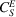) representing the converse piezoelectric effect is the transpose of matrix (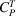) representing the piezoelectric effect. It will be shown in Section 1.1.3.4that they are the components of tensors of rank 3.